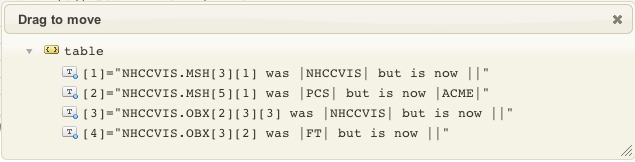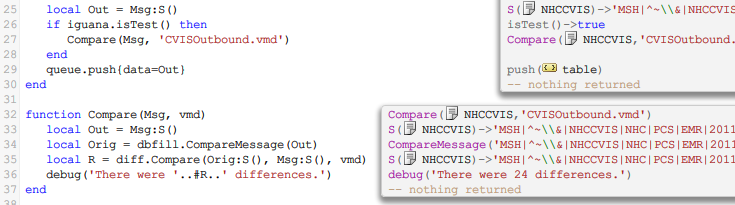# Database to HL7

## Contents

One of the powerful things about the Translator is the ability to traverse messages as “node trees” programmatically.

This makes it possible to write expressive code which can list differences between HL7 messages in a convenient manner where you can see the results in real time.

That’s exactly what I did for the next phase. I tweaked the dbfill.CompareMessage routine to obtain the original message and parse it into an HL7 node tree:

```local function LoadMessage(Base, Msg)
local FileName = 'D:temp'..Base
..Msg.MSH..'.txt'
trace(FileName)
local F = io.open(FileName, "r")
end

function dbfill.CompareMessage(Data)
local Result = nil
local Out = hl7.parse{vmd='CVISOutbound.vmd', data=Data}
if (Orig ~= Data) then
Result = 'Original and current messages differ!'
end
return hl7.parse{vmd='CVISOutbound.vmd', data=Orig}, Result, Orig
end```

And then I wrote a utility module called ‘diff’ would returns a nice human readable list of differences between the two trees like this:This made it very easy to locate the last few differences between the old interface and the new interface. The dialog would update in real time as I corrected the mappings. Here’s how it was called from the mapping routine:There was still one small difference which my diff routine did not detect – this is because the older interface was appending a constant to a subfield using python whereas the vmd had it configured as a single field. The difference showed up when comparing the HL7 messages directly.

To resolve that problem would require a tweak to the vmd file.

Here’s the source code for the diff module, you need to create the diff module and paste this code into it.

Here’s the mapping file after the last set of changes:

```require 'dateparse'
require 'node'
require 'dbfill'
require 'diff'

local conn = db.connect{
api=db.SQLITE,
name='test',
live=true
}

local function trace(a,b,c,d) return end

function main(Data)
local R = conn:query{
live=true,
sql="SELECT * FROM NHCCVISREPORT WHERE MESSAGE_ID = "..Data
}

local Msg = hl7.message{vmd='CVISOutbound.vmd', name='NHCCVIS'}

if #R == 0 then
print("Odd - unable to find message matching this one.")
return
end

MapMSH(Msg.MSH, R)
MapPID(Msg.PID, R)
MapPV1(Msg.PV1, R)
MapOBR(Msg.OBR, R)
MapOBX(Msg.OBX, R)
local Out = Msg:S()
if iguana.isTest() then
Compare(Msg, 'CVISOutbound.vmd')
end
queue.push{data=Out}
end

function Compare(Msg)
local Out = Msg:S()
local Orig = dbfill.CompareMessage(Out)
local R = diff.Compare(Orig:S(), Msg:S(), vmd)
trace('There were '..#R..' differences.')
end

function MapMSH(MSH, T)
MSH='ORU'
MSH='R01'
MSH = 'NHCCVIS'
MSH = 'NHC'
MSH = 'PCS'
MSH = 'EMR'
MSH = '2.3'
MSH = T.MSHMessageControlID
MSH = T.MSHDateTimeofMessage:HT()
MSH = 'T'
return MSH
end

function node.HD(N)
return os.date('%Y%m%d', dateparse.parse(N:S()))
end

function node.HT(N)
return os.date('%Y%m%d%H%M%S', dateparse.parse(N:S()))
end

function MapPID(PID, T)
PID = T.PIDPatientIDExternalIDID
PID = '11'
PID = 'EMI Primary'

PID = T.PIDPatientIDInternalIDID
PID = T.PIDPatientIDInternalIDCheckDigit
PID = '11'
PID = 'MRN'

PID = T.PIDPatientNameFamilyName
PID = T.PIDPatientNameGivenName
PID = T.PIDDateTimeofBirth:HD()
PID = T.PIDSex
PID = T.PIDPatientAccountNumberID
PID = "Visit"

return PID
end

function MapPV1(PV1, T)
PV1 = 'OUTPATIENT'
PV1 = 'NHCCVIS Assign'
PV1 = 'NHC'
PV1 = 'NA'
PV1 = T.PVVisitNumberID
PV1 = 'Visit'
end

function MapOBR(OBR, T)
OBR = 'PCS'
OBR = T.OBRFillerOrderNumberEntityIdentifier
OBR = 'NHCCVIS'
OBR = T.OBRUniversalServiceIDIdentifier
OBR = T.OBRUniversalServiceIDText
OBR = 'NHCCVIS'
OBR = T.OBRObservationDateTime:HT()
OBR = T.OBRPlacerField
OBR = 'F'
OBR = 'ROUTINE'
return OBR
end

function MapOBX(OBX, T)
OBX = '1'
OBX = 'FT'
OBX = T.OBXObservationIdentifierIdentifier
OBX = 'NHCCVIS'
OBX = T.OBXObservationValue
OBX = 'F'
OBX = '2'
OBX = 'RP'
OBX = T.OBXObservationValue1
OBX = T.OBXObservationIdentifierIdentifier1
OBX = 'NHCCVIS'
OBX = 'F'

OBX = '3'
OBX = 'FT'
OBX = T.OBXObservationIdentifierIdentifier2
OBX = T.OBXObservationIdentifierText2
OBX = 'NHCCVIS'
OBX = T.OBXObservationValue2
OBX = 'F'
end```

## Next Step?

The final step is make our last tweaks to code and the VMD file. However before that we will show the the source code for the diff module.